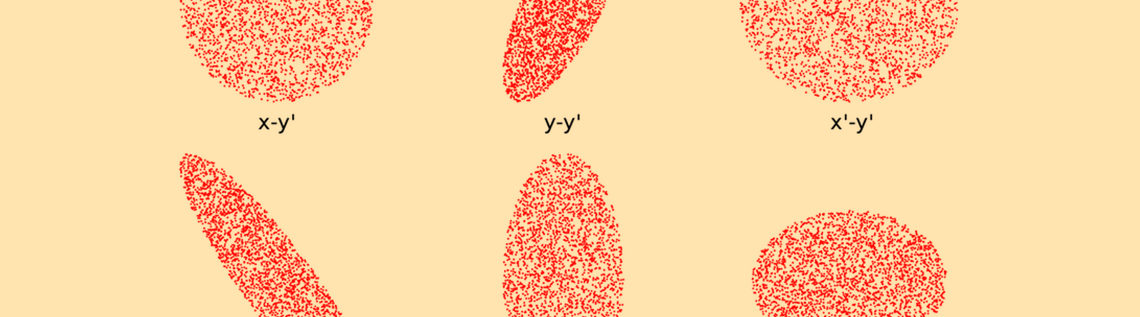# 三维带电粒子束的相空间分布取样

2016年 9月 22日

### 回顾椭圆形二维相空间分布

(1)

\gamma x^2 + 2\alpha x x' + \beta x'^2 = \varepsilon

(2)

\gamma \beta -\alpha^2 = 1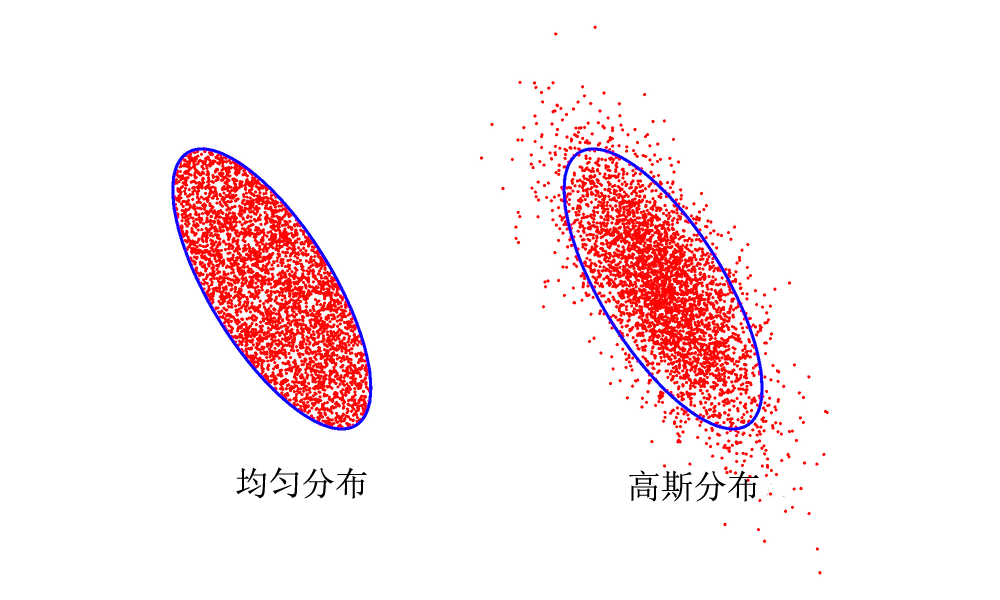(3)

x' = -\frac{\alpha x}{\beta} \pm \frac{\sqrt{\varepsilon \beta -x^2}}{\beta}

(4)

f(x,x') = \left\{
\begin{array}{cc}
C & -\frac{\alpha x}{\beta} -\frac{\sqrt{\varepsilon \beta -x^2}}{\beta} \textless x' \textless -\frac{\alpha x}{\beta} + \frac{\sqrt{\varepsilon \beta -x^2}}{\beta}\\
0 & \textrm{otherwise}
\end{array}\right.

g(x) = \int_{-\infty}^{\infty} f(x,x')dx'

g(x) = \left\{
\begin{array}{cc}
2C \frac{\sqrt{\varepsilon \beta -x^2}}{\beta} & -\sqrt{\varepsilon \beta} \textless x \textless \sqrt{\varepsilon \beta}\\
0 & \textrm{otherwise}
\end{array}\right.

(5)

g(x) \propto \frac{\sqrt{\varepsilon \beta -x^2}}{\beta}, \qquad -\sqrt{\varepsilon \beta} < x < \sqrt{\varepsilon \beta}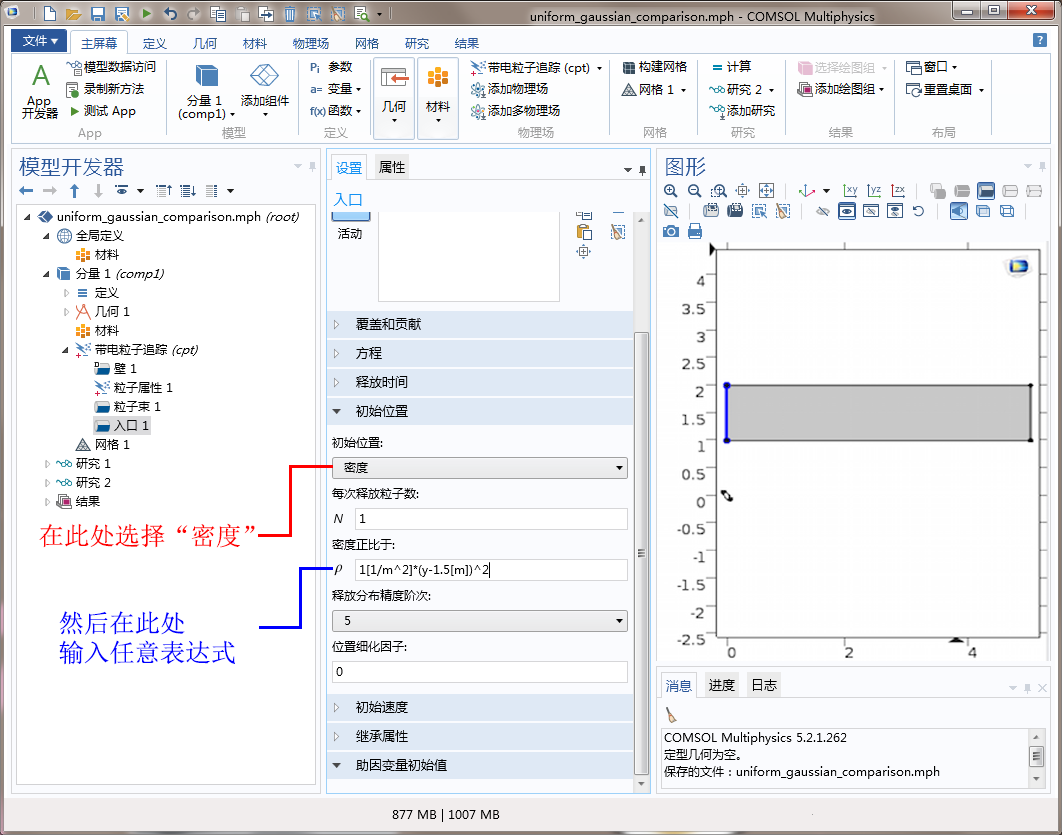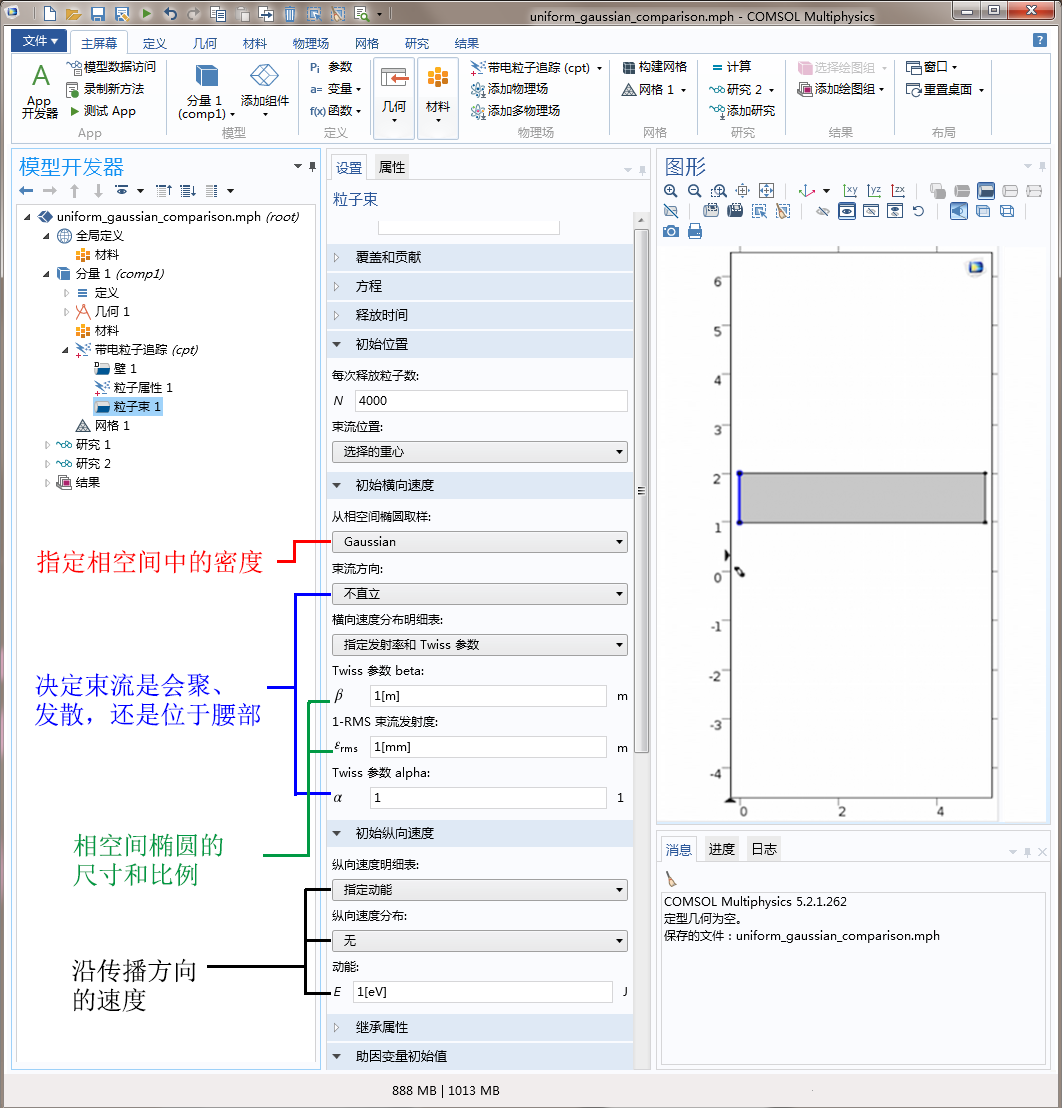### 模拟三维带电粒子束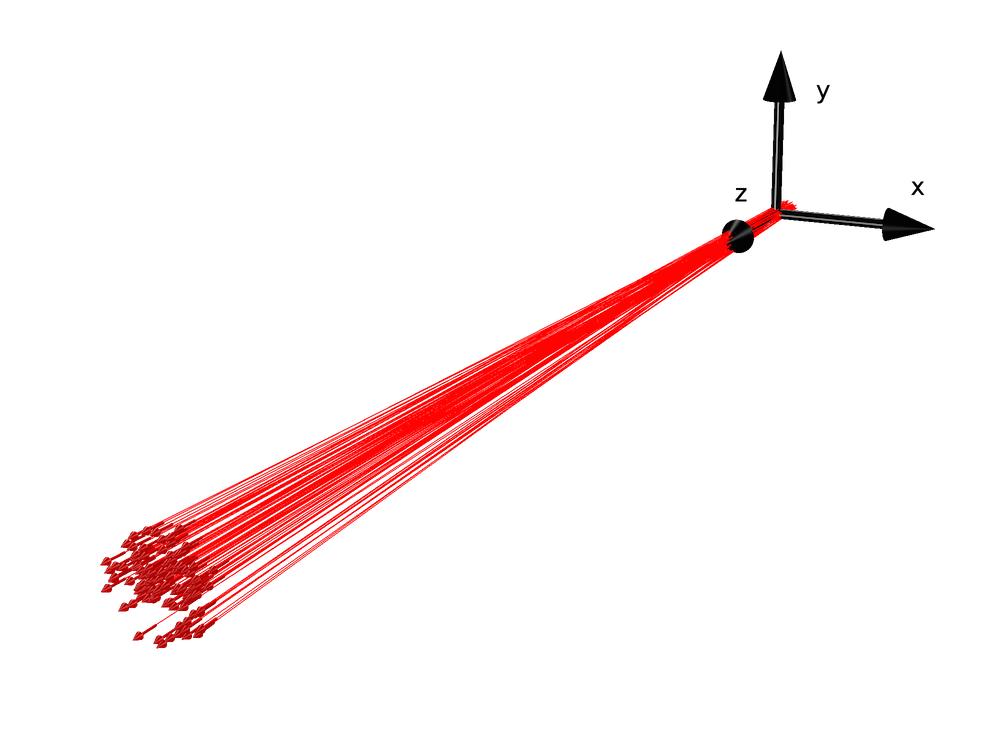\left(\frac{x}{r_x} \right)^2
+\left(\frac{r_x x' -r'_x x}{\varepsilon_x} \right)^2
+\left(\frac{y}{r_y} \right)^2
+\left(\frac{r_y y' -r'_y y}{\varepsilon_y} \right)^2 = 1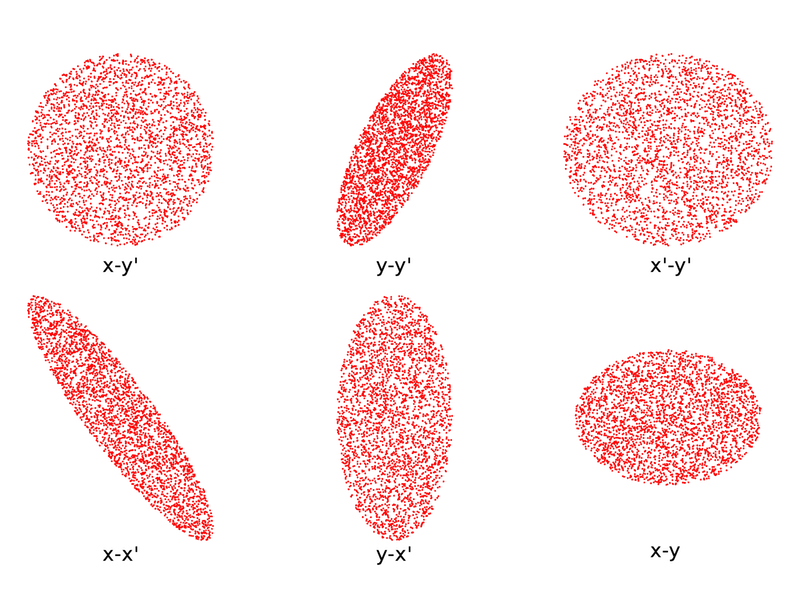### 参考文献

1. Humphries, Stanley. Principles of charged particle acceleration. Courier Corporation, 2013.
2. Humphries, Stanley. Charged particle beams. Courier Corporation, 2013.
3. Davidson, Ronald C., and Hong Qin. Physics of intense charged particle beams in high energy accelerators. Imperial college press, 2001.
4. Lund, Steven M., Takashi Kikuchi, and Ronald C. Davidson. “Generation of initial Vlasov distributions for simulation of charged particle beams with high space-charge intensity.” Physical Review Special Topics — Accelerators and Beams, vol. 12, N/A, November 19, 2009, pp. 114801 12, no. UCRL-JRNL-229998 (2007).
5. Lund, Steven M., Takashi Kikuchi, and Ronald C. Davidson. “Generation of initial kinetic distributions for simulation of long-pulse charged particle beams with high space-charge intensity.” Physical Review Special Topics — Accelerators and Beams, 12, no. 11 (2009): 114801.
6. Batygin, Y. K. “Particle distribution generator in 4D phase space.” Computational Accelerator Physics, vol. 297, no. 1, pp. 419-426. AIP Publishing, 1993.
7. Batygin, Y. K. “Particle-in-cell code BEAMPATH for beam dynamics simulations in linear accelerators and beamlines.” Nuclear Instruments and Methods in Physics Research. Section A: Accelerators, Spectrometers, Detectors and Associated Equipment 539, no. 3 (2005): 455-489.

#### 评论 (2)

##### 留言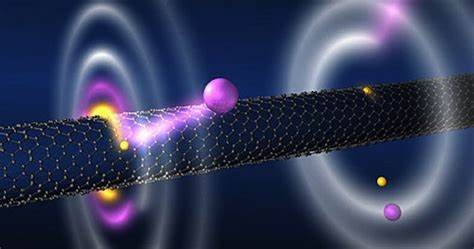##### zang retain
2020-04-23

can you send me some tutorial of charged ion motion in electromagnetic filed please ?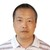##### 王 刚
2020-05-14 COMSOL 员工

There some demo examples in Applications Library shipped with COMSOL Multiphysics installation. You can also find these examples in Applications Gallery, e.g., http://cn.comsol.com/models/particle-tracing-module?q=%E7%94%B5.